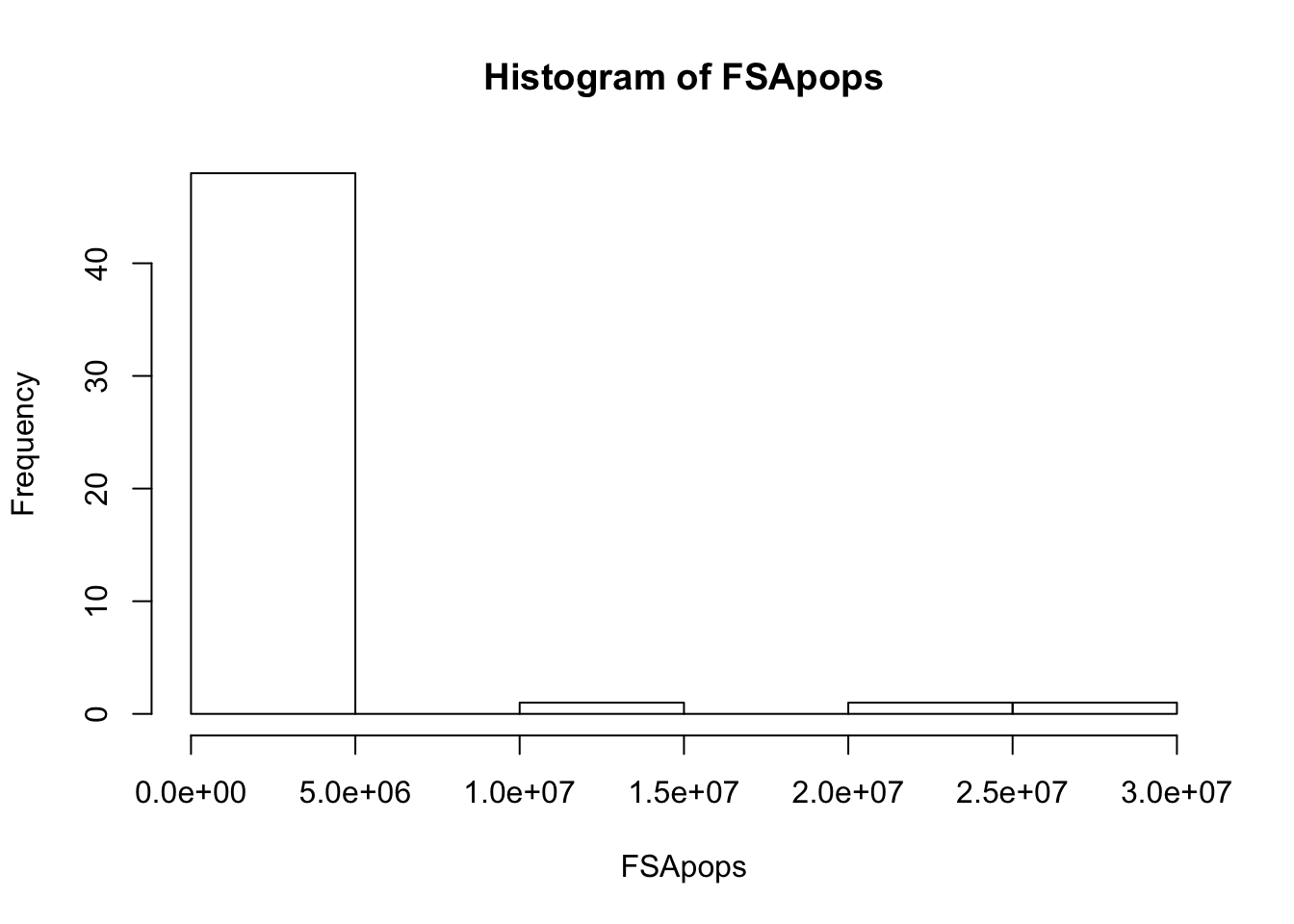## Task 1: Write, test, and submit the necessary code in R

 # 1. Generate a normal distribution, or 1000 samples, with a mean of 80
Distribution <- rnorm(1000, 80)

# 2. Write a function
# create a function called "myFunction" that takes three variables
myFunction <- function(vector, minimum, maximum)
{
# only keep the elements within the vector that are between the min and max, and store the number of eligible elements into the variable "count"
count <- length(vector[vector >= minimum & vector <= maximum])
# calculate the percentage and return the results
return(count/length(vector))
}

# test the function
myFunction(c(1,3,5,7,9,11), 2, 9)
##  0.6666667
 # 3. Use the function to see how many of your normal distribution samples are within the range of 79 to 81
# pass the "distribution" as the vector parameter, 79 as the minimum parameter, and 81 as the maximum parameter
myFunction(Distribution, 79, 81)
##  0.679
  # 4. Repeat 3 times, to see if the results vary
# The results vary because everytime rnorm() would generate a different set of 1000 numbers.
myFunction(rnorm(1000, 80), 79, 81) 
##  0.667
 myFunction(rnorm(1000, 80), 79, 81)
##  0.696
 myFunction(rnorm(1000, 80), 79, 81)
##  0.711

## Task 2: Write, test, and submit the necessary code in R

install.packages(“VGAM”)

#install.packages("VGAM")
library(VGAM)
## Warning: package 'VGAM' was built under R version 3.5.2
## Loading required package: stats4
## Loading required package: splines
# 1 & 2
# rpareto(n, m, s): generating random numbers that fit a Pareto distribution
# n -- generate 51 values; m -- location parameter (set it to be about the population size of Wyoming);
# s -- vector of dispersion parameters.
FSApops <- rpareto(51, 560000, 1)
# As you increase s mean decreases...values deviate from the mean, but higher SD (since its more dispersed)

# 3. create a histogram that shows the distribution of state populations
hist(FSApops)# 4. Report the actual mean and standard deviation of the 51 values stored in FSApops.
mean(FSApops)
##  2448107
 sd(FSApops)
##  5086701
 # 5. Use a command to report the population of your largest fictional state and your smallest fictional state
min(FSApops)
##  566433.4
 max(FSApops)
##  28709338